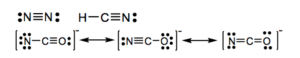Chapter 9. Chemical Bonding and Lewis Structures

# 9.7 End of Chapter Problems

1. List 5 ions that are isoelectronic with Kr.

2. Determine the electron configuration for the following ions: a)  Pd2+     b) Rh3+     c)  Ca2+     d) S2-

3. If carbon formed a stable ion, what charge would you expect it to have?  Explain.

4. Explain the differences and similarities between covalent bonds, ionic bonds and polar covalent bonds.

5. Given that ions in LiF and MgO are of similar size, which compound has stronger ionic bonding?  Use Couloumb’s law to explain your answer.

6. How can ionic compounds be neutral if they contain positive and negative ions?

7. Are ions present in a sample of NH3?  Explain.

8. Are molecules present in a sample of KBr? Explain.

9. The radii of sodium and potassium ions are 102 pm and 138 pm respectively. Which compound has stronger ionic attractions, sodium chloride or potassium chloride?

10. An ionic compound forms when lithium (Z = 3) reacts with oxygen (Z = 8). If a sample of the compound contains 5.3 x 1020lithium ions, how many oxide ions does it contain?

11. Explain the difference between electronegativity and ionization energy. What is the general periodic table trend for each? How do we use that trend to predict bond characteristics?

12. For each of the following bonds, draw a dipole moment (if it is present) and show the partial charges. Hint: Just focus on the periodic table trend.

a) C-F      b)   N-O    c)   Si-F     d)  Si-N

13. Without using actual electronegativity values, predict which bond in #12 above will have the most ionic character. Do the electronegativity values support your statement?  Explain.

14. Write the Lewis dot diagram for the following:

a)  SiF4            b) NBr3           c)  PO43-         d) CO               e)  NO3

15. Give Lewis electron dot symbols for:

a)  Mg              b)  Mg2+           c) I                 d) Te                e)  Ga

16. Draw electron dot diagrams for the following compounds. If the compound is ionic, give the dot symbols for the ions separately, so as not to imply that the ions are sharing electrons.  (In a group of atoms, the central atom is underlined.)

a)  OCl2           b) MgCl2          c)  CF4             d)  HCN            e)SO2             f)  BaO            g)  H2S

17. Give electron dot diagrams for:

a)  PO43-          b)  NH4+          c) ClO2           d)  SO32-

18. Give electron dot diagrams for the following. If more than one possible structure satisfies the rules, write ALL possible structures:

a)  N2               b)  HCN            c)  NCO

1.   Se2-,  Br,  Rb+,  Sr2+,  Y3+

2.   a) [Kr]4d8;   b) [Kr]4d6;   c) [Ar];  d) [Ar]

3.   C4+or C4-. To reach the next noble gas configuration, which is very stable.

4.   All bonds are a force that holds atoms together that results from the attraction of ions to the nuclei of atoms. In ionic bonds, electrons are donated from the metal to the nonmetal. In a covalent bond, electrons are shared between two identical non metals. In a polar covalent bond, electrons are unequally shared between two different non metals.

5.   If the sizes are the same, the bond strength will depend only on the charges (strength is proportional to q1x q2). Mg and O both have charges with the magnitude of 2 (+2 and -2), whereas Li and F have charges of 1 (+1, -1). Therefore the strength of the MgO bond will be stronger than the LiF bond.

6.   Ionic compounds are neutral overall. The total negative charge of the anions is balanced by the total positive charge of the cations.

7.   No. NH3is a covalent compound made of N and H (both non-metal). Even though it would have polar covalent bonds (and thus partial positive and negative charges) this does not make them ions.

8.   No. KBr is an ionic compound and exists as an ionic solid, not as individual molecules. KBr is better described as a formula unit that represents the overall composition of the ionic solid.

9.   According to Couloumb’s Law, the strength of a bond is inversely proportional to the distance between atoms. If sodium is smaller, the distance between the centre of sodium and the centre of chloride will be smaller, therefore the bond strength will be greater as compared to KCl.

10.  Li+and O2-therefore compound formed would be Li2O. Thus there are half as many oxide ions compared to Li ions, therefore 2.7 x 1020ions.

11.  Ionization energy is the ability of an atom’s nucleus to attract one of the atoms own electrons, whereas electronegativity (EN) is the relativity ability of an atom in a molecule to attract the shared electrons in a chemical bond.
The general trend for both IE and EN are the same: they increase bottom to top and right to left.
The difference in EN values determines the polarity of a bond, therefore the further apart the two atoms are on the periodic table, the more polar the bond will be.

12.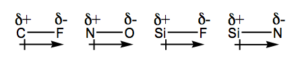13.  Si—F is the most polar bond.
ΔEN (C—F) = 4.0 – 2.5 = 1.5
ΔEN (N—O) = 3.5 – 3.0 = 0.5
ΔEN (Si—F) = 4.0 – 1.8 = 2.2
ΔEN (Si—N) = 3.0 – 1.8 = 1.2
The larger the the difference in EN values, the more polar the bond.

14.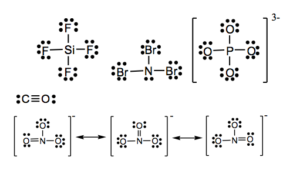15.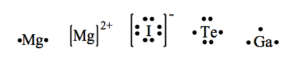16.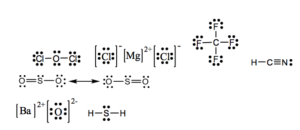17.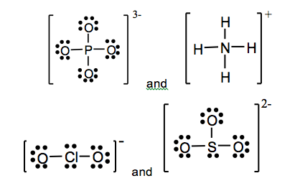18.